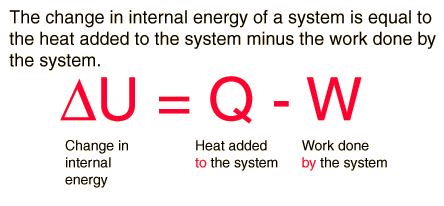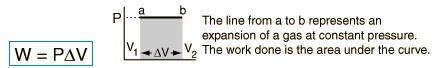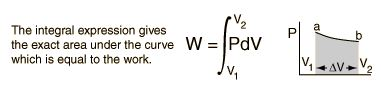Courses

# Enthalpy - Thermodynamic and Statistical Physics, CSIR-NET Physical Sciences Physics Notes | EduRev

## Physics for IIT JAM, UGC - NET, CSIR NET

Created by: Akhilesh Thakur

## Physics : Enthalpy - Thermodynamic and Statistical Physics, CSIR-NET Physical Sciences Physics Notes | EduRev

The document Enthalpy - Thermodynamic and Statistical Physics, CSIR-NET Physical Sciences Physics Notes | EduRev is a part of the Physics Course Physics for IIT JAM, UGC - NET, CSIR NET.
All you need of Physics at this link: Physics

First Law of Thermodynamics

The first law of thermodynamics is the application of the conservation of energy principle to heat and thermodynamic processes:The first law makes use of the key concepts of internal energy, heat, and system work. It is used extensively in the discussion of heat engines. The standard unit for all these quantities would be the joule, although they are sometimes expressed in calories or BTU.

It is typical for chemistry texts to write the first law as ΔU=Q+W. It is the same law, of course - the thermodynamic expression of the conservation of energy principle. It is just that W is defined as the work done on the system instead of work done by the system. In the context of physics, the common scenario is one of adding heat to a volume of gas and using the expansion of that gas to do work, as in the pushing down of a piston in an internal combustion engine. In the context of chemical reactions and process, it may be more common to deal with situations where work is done on the system rather than by it.

Enthalpy

Four quantities called "thermodynamic potentials" are useful in the chemical thermodynamics of reactions and non-cyclic processes. They are internal energy, the enthalpy, the Helmholtz free energy and the Gibbs free energy. Enthalpy is defined by

H = U + PV

where P and V are the pressure and volume, and U is internal energy. Enthalpy is then a precisely measurable state variable, since it is defined in terms of three other precisely definable state variables. It is somewhat parallel to the first law of thermodynamics for a constant pressure system

Q = ΔU + PΔV since in this case Q=ΔH

It is a useful quantity for tracking chemical reactions. If as a result of an exothermic reaction some energy is released to a system, it has to show up in some measurable form in terms of the state variables. An increase in the enthalpy H = U + PV might be associated with an increase in internal energy which could be measured by calorimetry, or with work done by the system, or a combination of the two.

The internal energy U might be thought of as the energy required to create a system in the absence of changes in temperature or volume. But if the process changes the volume, as in a chemical reaction which produces a gaseous product, then work must be done to produce the change in volume. For a constant pressure process the work you must do to produce a volume change ΔV is PΔV. Then the term PV can be interpreted as the work you must do to "create room" for the system if you presume it started at zero volume.

System Work

When work is done by a thermodynamic system, it is ususlly a gas that is doing the work. The work done by a gas at constant pressure is:For non-constant pressure, the work can be visualized as the area under the pressure-volume curve which represents the process taking place. The more general expression for work done is:Work done by a system decreases the internal energy of the system, as indicated in the First Law of Thermodynamics. System work is a major focus in the discussion of heat engines.

Offer running on EduRev: Apply code STAYHOME200 to get INR 200 off on our premium plan EduRev Infinity!

159 docs

,

,

,

,

,

,

,

,

,

,

,

,

,

,

,

,

,

,

,

,

,

,

,

,

;